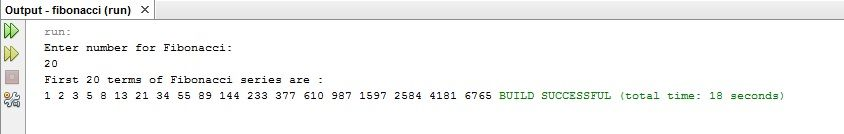Heyaaaaaa!  Advance Happy  Halloween everyone! Since its my semestral break A.K.A SEMBREAK just like before, I’m going to post some of my programming codes here which was taken previous semester.. here ya go.

Purpose: To display the “First 20 terms of Fibonacci Series.”

Source Code:

package fibonacci;

import java.util.*;
/**
*
* @author Christine Cloma
*/
public class Fibonacci {

/**
* @param args the command line arguments
*/
public static void main(String[] args) {
Scanner console = new Scanner (System.in);
int n, c, first = 0, second = 1, next;

System.out.println( “Enter number for Fibonacci: “);
n = console.nextInt();

System.out.println(“First ” + n + ” terms of Fibonacci series are : ” );

c = 1;
do
{
next = first + second;
first = second;
second = next;

System.out.print(next+ ” “);
c++;
}while(c<n);

}
}

PS: I used Netbeans IDE 8.0 version. 🙂

-tinay